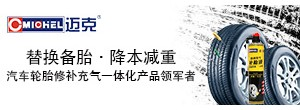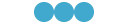# [分享] 注塑工程师必会！超实用的注塑工艺计算公式发表于 2020-9-27 15:02:29 | 显示全部楼层 |阅读模式
<

### 亲，赶快注册吧，有更多精彩内容分享！

x

【汽车材料网】注塑工程师必会！超实用的注塑工艺计算公式

1锁模力 F(TON) 公式F=Am*Pv/1000
F：锁模力:TON Am：模腔投影面积:CM2
Pv：充填压力:KG/CM2
(一般塑胶材料充填压力在150-350KG/CM2)
(流动性良好取较底值，流动不良取较高值)

2射出压力 Pi(KG/CM2)公式Pi=P*A/Ao

Pi: 射出压力 P：泵浦压力 A：射出油缸有效面积
Ao:螺杆截面积
A=π*D2/4 D：直径 π：圆周率3.14159

Pi=75*150/15.9=707 KG/CM2

3射出容积 V(CM3)公式V= π*(1/2Do)2*ST

V：射出容积 CM3 π：圆周率 3.1415 Do：螺杆直径 CM
ST：射出行程 CM

V= π*(4.2÷2)2*16.5=228.6CM3

4射出重量 Vw(g) 公式Vw=V*η*δ

Vw：射出重量 g V：射出容积 η：比重 δ：机械效率

5射出速度 S(CM/SEC)公式S=Q/A

S：射出速度 CM/SEC
A：射出油缸有效面积 CM2
Q：泵浦吐出量 CC/REV公式：Q=Qr*RPM/60 (每分钟/L)即：泵浦吐出量=泵浦每转吐出量*马达回转数/每分钟
Qr：泵浦每转吐出量(每回转/CC)
RPM：马达回转数/每分钟

S=85*1000/60/140=10.1 CM/SEC

6射出率 Sv(G/SEC)公式Sv=S*Ao

Sv：射出率G/SEC S：射出速度CM/SEC Ao：螺杆截面积

Sv=13.85*10=138.5G/SEC

1理论出容积:(π/4=0.785)(1)螺杆直径2*0.785*射出行程=理论射出容积(cm3);
(2)理论射出容积/0.785/螺杆直径=射出行程(cm).

2射出重量理论射出容积*塑料比重*射出常数(0.95)理想=射出重量(gr);

3射出压力(1)射出缸面积2/螺杆面积2*系统最大压力(140kg/cm2)2=射出压力(kg/cm2);
(2)射出缸直径2/螺杆直径2*系统最大压力(140kg/cm2)=射出压力(kg/cm2);
(3)料管组合最大射出压力*实际使用压力(kg/cm2)/系统最大压力(140kg/cm2)=射出压力(kg/cm2).

4射出速率(1)螺杆面积(cm2)*射出速度(cm/sec)=射出速率(cm3/sec);
(2)螺杆直径(cm2)*0.785*射出速度(cm/sec)=射出速度(cm3/sec).

5射出速度(1)射出速率(cm3/sec)/螺杆面积(cm2)=射出速度(cm/sec);
(2)泵浦单转容积(cc/rev)*马达转速(rev/sec)/60(秒)/射出面积(cm2)=射出速度(cm/sec).
(马达转速RPM:60HZ------1150,50HZ-----958)

6射出缸面积(1)射出压力(kg/cm2)/系统最大压力(140kg/cm2)*料管面积(cm2)=射出缸面积(cm2);
(2)单缸---(射缸直径2-柱塞直径2)*0.785=射出缸面积(cm2);

7泵浦单转容积射出缸面积(cm2)*射出速度(cm/sec)*60秒/马达转速=泵浦单转容积(cc/sec)，(马达转速RPM: 60HZ------1150,50HZ-----958)

8螺杆转速及油压马达单转容积(1)泵浦单转容积(cc/rec)*马达转速(RPM)/油压马达单转容积=螺杆转速;
(2)泵浦单转容积(cc/rec)*马达转速(RPM)/螺杆转速=油压马达单转容积.

9射出总压力(1)系统最大压力(kg/cm2)*射出缸面积(cm2)=射出总压力(kg);
(2)射出压力(kg/cm2)*螺杆面积(cm2)=射出总压力(kg).

10盎司及相关单位换算(1)1盎司(oz)=28.375公克(gr);
(2)1磅(ib)=16盎司(oz);
(3)1公斤(kg)=2.2磅(ib);即：1斤=1.1磅;
(4)1磅(ib)=454公克(gr)=0.454公斤(kg).

11关模力(1)曲手式:

(2)单缸直压式:

12道柱直径和关模力的公式道柱直径2(cm2)*0.785*杨氏系数(scm4约1000kg/cm2)*4=关模力概值(Ton).

13成品排列投影面积和关模力关系公式成品排列投影面积(寸2)*标准厚度(1.5mm)/成品平均厚度(mm)*使用原料常数/PS原料常数(1)=关模力(Ton);
(1)成品排列投影面影以射入浇口为圆心,长边为半径计算出直径;排列直径2(寸2)*0.785=成品排列投影面积(寸2)
(2)使用原料常数,以概略经验值计算,以流动性良劣比PS好的列为1以下;比PS不好的列为1以上.如:

ABS 1.05; AS 1.2; PMMA 1.3; PC 1.6; PBT 0.9;
PP 0.7; PE 0.7-0.8;塑胶钢 0.8; NILON 0.7-0.9¨¨¨等

(3)和射出从向的成品部份之要求,如杯子的高度部份大约以投影面积的30%计算即可

14托模力托模缸面积(cm2)*系统最大压力(140kg/cm2)/1000=托模力(Ton)

15电力单位1马力(HP)=0.754千瓦(KW);
1千瓦(KW)=1.326马力(HP)=1000瓦(W);
1千瓦(KW)=1度电计量单位(1KW/Hr).

16泵浦大小和马力关系P=最大使用压力(如:125kg/cm2.140kg/cm2);
Q=油泵浦一分钟吐出量(L/min);
Q=油泵浦单转容积(cc/rec)*马达转速(RPM)/1000=油泵浦一分钟吐出量(L/min).

P*Q/540=HP;
P*Q/612=KW.

HP*450/Q=LP;
KW*612/Q=LP

17计算使用电力(马达容量+电热容量+烘干机容量)*用电常数(约40%)=实际每小时用电量(度,KW/Hr)1什么是注塑机的射出能力?射出能力=射出压力(kg/cm2)×射出容积(cm3)/1000

2什么是注塑机的射出马力?射出马力PW(KW)=射出压力(kg/cm2)×射出率(cm3/sec)×9.8×100%

3什么是注塑机的射出率?射出率V(cc/sec)=p/4×d2×g
d2：：料管直径 g：料的密度

4什么是注塑机的射胶推力?射胶推力F(kgf)=p/4(D12-D22)×P×2
D1：油缸内径 D2：活塞杆外径 P：系统压力

5什么是注塑机的射胶压力?射胶压力P(kg/cm2)=[p/4×(D12-D22)×P×2]/(p/4×d2)

6什么是注塑机的塑化能力?塑化能力
W(g/sec)=2.5×(d/2.54)2×(h/2.54)×N×S×1000/3600/2
h=螺杆前端牙深(cm) S=原料密度

7什么是系统压力?与注塑压力有什么区别?系统压力(kg/cm2)=油压回路中设定最高的工作压力

8射出速度?H：速度=距离/时间

### 相关帖子

 本版积分规则 回帖并转播 回帖后跳转到最后一页